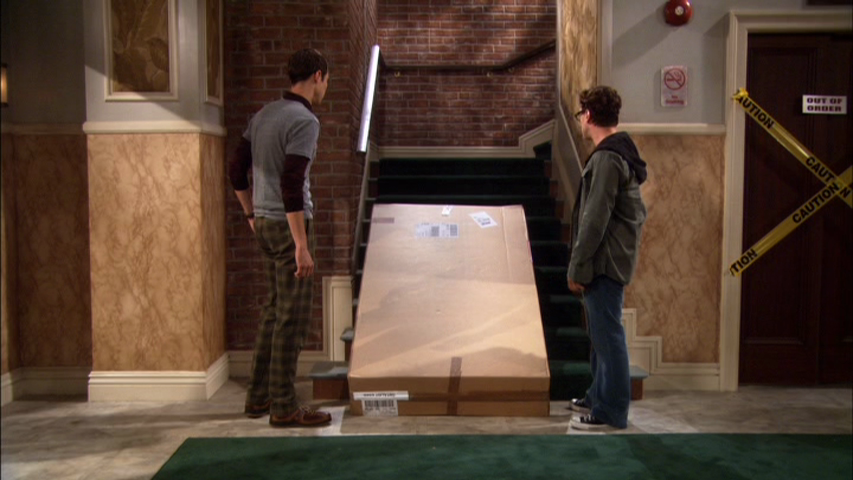# Trecherous inclineTwo friends decide to shove boxes up a rough plank inclined at an angle of $\theta$. The plank is slightly smoother at the bottom and a bit rougher at the top, such that the coefficient of kinetic friction increases linearly with the distance $s$ along the plank:$\mu_k=ks$. One of the friends shoves a box up the plank so that it leaves the bottom of the plank at a speed of $V_{0}$. Assuming that the coefficients of kinetic and static friction are equal $\mu_k=\mu_s$, when the box first comes to rest, it will remain at rest if $(V_{0})^A \geq \frac{B \times g \times sin^C(\theta)}{k \times cos^D(\theta)}$ For some constant positive integers $A,B,C,D$; what is the value of $A+B+C+D$?

Details and Assumptions

• $g$ is gravitational acceleration
×